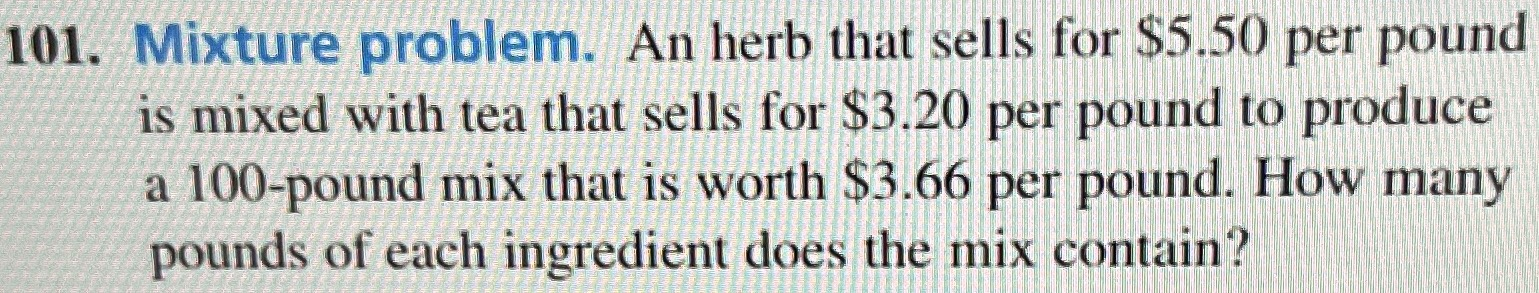### ¿Todavía tienes preguntas de matemáticas?

Pregunte a nuestros tutores expertos
Algebra
Pregunta101. Mixture problem. An herb that sells for $$\ 5.50$$ per pound is mixed with tea that sells for $$\ 3.20$$ per pound to produce a $$100$$ -pound mix that is worth $$\ 3.66$$ per pound. How many pounds of each ingredient does the mix contain?